Outer sums and products

To combine tensors in an outer sum, they have to be of the same rank. Thus we may combine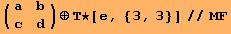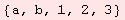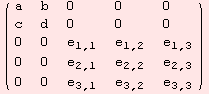We may also take the outer sum of two ad tensors that define Lie algebras.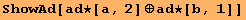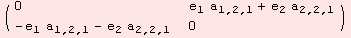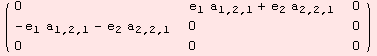Tensors of any rank are eligible to be combined in an outer product. Examples are given below.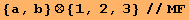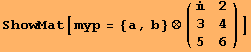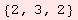As a matter of definition - and in fact recursion - the tensor product between a factor x∈R an a tensor X simply is the multiple x of the tensor.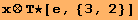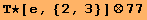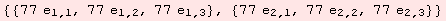Created by Mathematica  (September 30, 2006)